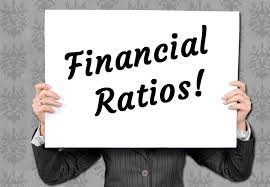In my last post, we already started the discussion on Activity ratio and we discussed average collection period already. In addition to what I already said about average collection period, it is a good business principle to have a shorter collection period and a longer payment period. That is, you receive money faster from your debtor or account receivables while you pay your own creditors much later. This will help to ensure more liquidity in the company. Now, let us continue with the financial ratio analysis.

Another type of activity ratio that is use in financial analysis is account receivable turnover. You know the number of times it takes to convert account receivable to sales in a year or period. It thus show how much the company is able to manage its credit. The formula: divide turnover by account receivable.

The third ratio in this category is average payment period. This is similar to average collection period. The only difference here is that average payment period calculates how many days it takes to pay creditors. The longer, the better. The formula: account payable divided by account purchases or cost of sales.

## PROFITABILITY RATIO ANALYSIS

This ratio category the performance of the company in respect to profitability. It includes: return on asset, gross profit margin, return on equity and so on.

Gross profit margin calculates the proportion of sales that makes up the gross profit. Simply put, express gross margin as a percentage of sales. That is gross margin divided by sales. It shows the efficiency of the company in generating profit for every amount of cost involved. The higher it is, shows how much the management managed cost of sales.

The second ratio in this category is return on asset or return on capital employed. As the name implies, we want to know what we earn in return for the resources used in generating income. The ratio thus, shows relative profitability of the company. Earnings before interest and tax divided by total assets.

Return on equity also falls in this category. It calculates the amount of profit attributable to shareholders fund. It is an indication of profitability. To calculate it, divide profit after tax and preference dividend by shareholders’ equity and multiply by 100%.

## INVESTORS’ RATIO

1. Earning per share: You can use this ratio to ascertain profitability as well as investor’s ratio. It simply tells how much each share earn from the net income. It is an indicator of profitability and growth in the worth of the shares held. It is always in kobo.
2. Dividend per share: Sometimes, it is not all the profit or net income made is declared as dividend. Some part of the profit are retained or returned back to the business; while the remaining are dividend. To get dividend per share, divide dividend paid to ordinary shareholders by number of ordinary share holders.
3. Price Earnings: It shows the number of times the earnings per share is covered by the current market price of the stock. The lower, the price earnings ratio, the better. It shows the stock is valuable.

## SOLVENCY RATIO

This ratio is similar to liquidity only that this focus on long term obligations while liquidity focus on short term obligations. Examples are gearing ratio, debt equity ratio and so on.## Next Generation Fixed Wireless Access (NGFWA) for Service Providers: A Viable Alternative to Traditional Fiber-Optic Networks

in## Transforming Nigeria’s Local Government System for Rural Economic Growth and Increased GDP

in